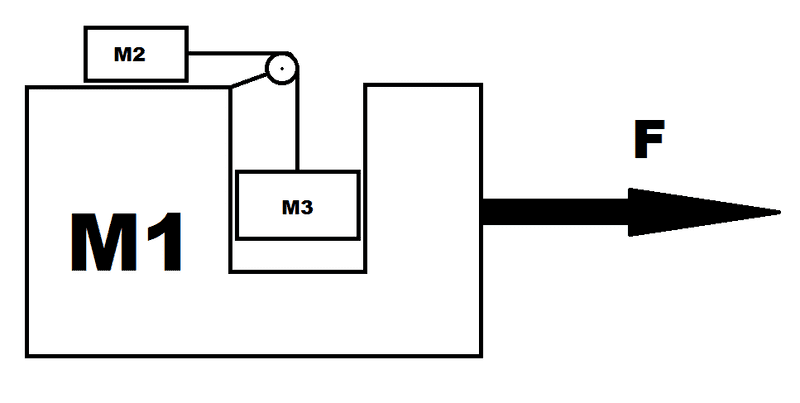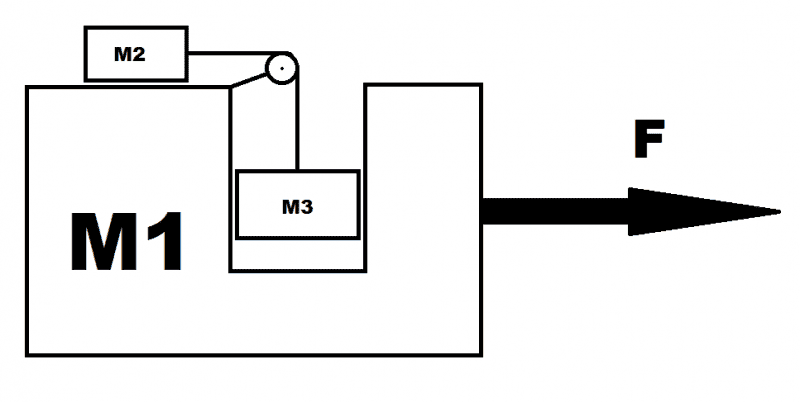# Pedagogical machine problem (K&K 2.20)

## Homework Statement

For context, the first question for this diagram asks for the force F on m1 needed to keep m3 at a constant height.
The second question (which I'm struggling with) asks for the acceleration of m1 in the case where the force F=0.
All surfaces are frictionless and I've assumed the rope is massless## The Attempt at a Solution

I'm not sure I even understand the question - are we to find the acceleration of m1 in the case where m3 is at a constant height as in the first part?
If so, how can m1 accelerate if there is no force on it?

haruspex
Homework Helper
Gold Member
2020 Award
I the second part m3 is not at constant height.

SammyS
Staff Emeritus
Homework Helper
Gold Member

## Homework Statement

For context, the first question for this diagram asks for the force F on m1 needed to keep m3 at a constant height.
The second question (which I'm struggling with) asks for the acceleration of m1 in the case where the force F=0.
All surfaces are frictionless and I've assumed the rope is massless

[ IMG]http://i.stack.imgur.com/8OXPf.png[/PLAIN]

## The Attempt at a Solution

I'm not sure I even understand the question - are we to find the acceleration of m1 in the case where m3 is at a constant height as in the first part?
If so, how can m1 accelerate if there is no force on it?

Hello wesson. Welcome to PF .

(Use the X2 icon for subscripts.)

In the first part you found the force, F, needed to keep m3 at a constant height. That force was not zero, was it?

In the second part, the applied force, F, is zero. The horizontal component of the net force on the system is zero, but the net force on m1 is not zero.

m1, m2, and m3 all accelerate.Stephen Tashi
If so, how can m1 accelerate if there is no force on it?

The mass M1 wouldn't accelerate in the horizontal direction if there is no net force on it in that direction. But F isn't the net force on m1 in the horizontal direction. The tension of the string causes a horizontal component of force on M1. (You can imagine the support holding the pulley as exerting a force on M1.)

I think you are supposed to imagine M3 still "in the air" but falling down - or perhaps you are supposed to give one answer that applies before M3 "hits bottom" and another answer after it lands.

Thanks for the replies, everyone.

How does the tension on the string exert a force on the pulley if it's massless and the surfaces are frictionless?

haruspex
Homework Helper
Gold Member
2020 Award
Thanks for the replies, everyone.

How does the tension on the string exert a force on the pulley if it's massless and the surfaces are frictionless?
The rope presses against the surface of the pulley.

Stephen Tashi
How does the tension on the string exert a force on the pulley if it's massless and the surfaces are frictionless?

"How?" can be a question about what physical mechanisms allows a string to exert the force on the pulley. That is not dealt wtih in such theoretical problems - and dealing with it would contradict "frictionless" and "massless" anyway.

"How" in the sense of "How am I supposed to know to assume that?" is not so simple to answer! I've never see a systematic treatment of the mechanics of frictionless and massless objects. Most people learn conventions about them from seeing worked examples. It would be useful to compile as set of sketches from such examples showing how various massless and frictionless contraptions transmit forces. (Using examples is easier than working out a theory rigorously. To do that, you'd have to set up equations that apply when there is mass and friction and then take the limit as mass and frictional forces approached zero.)

In this type of physics we pay attention to situations where an object with a mass (a "free body") is being accelerated. If it is being accelerated, it must have a non-zero net force on it. If it is not accelerating, the net force on it must be zero. There is no law in this type of physics that says two things in contact must exert a force on each other.

Between the bodies with mass there may be (theoretically) bodies with no mass. There may be surfaces that make contact with no friction. There is no theoretical realationship (from F = MA) between the net force on a massless body and its acceleration or the lack of it. So if we try to analyze a massless obect as a "free body" we are on shaky ground.

My personal way of approaching this situation is to imagine the massless objects as parts of an object that has mass. So I would imagine the support and the pulley as part of M1. Then I would simplify the pulley. Since the pully has no moment of inertia, I might as well imagine the pulley to be a fixed instead of turning give it frictionless surface for the string to slide over. If I do that then why not shrink the pulley down to a point on the end of the support. After that I have a situation resembling a bow and arrow. The support for the pulley is the arrow. I sense that even if the string of a bow is frictionless, it would exert a force on the end of the arrow. I know (from seeing example problems) that the tension on massless strings is the same at the end of any section of the string.

So I have two sections of string pushing with tension T on the support and thus on mass M1. Mass M1 is not accelerating in the vertical direction, so the net vertical component of force on M1 is zero. This tells me that the tension of the vertical part of the string plus the force of gravity on M1 is canceled out by the upward force of whatever surface is below M1. M1 could be accelerating in the horizontal direction. The net force in the horizontal direction is the tension from the horizontal part of the string.

My apologies for the delayed response.

I still can't see how the tension of the string is able to exert a horizontal force on M1 if there is no friction between them (specifically, the horizontal bit over the pulley). I'm not sure how the vertical bit of string would exert a force either because M3 is in the way.

And also, doesn't tension only act along the length of a string? How would this be the force on M1?

Stephen Tashi
Is it fair to say we can simplify your question to "How could a frictionless string on a bow exert force on an arrow?".

•billy_joule
haruspex